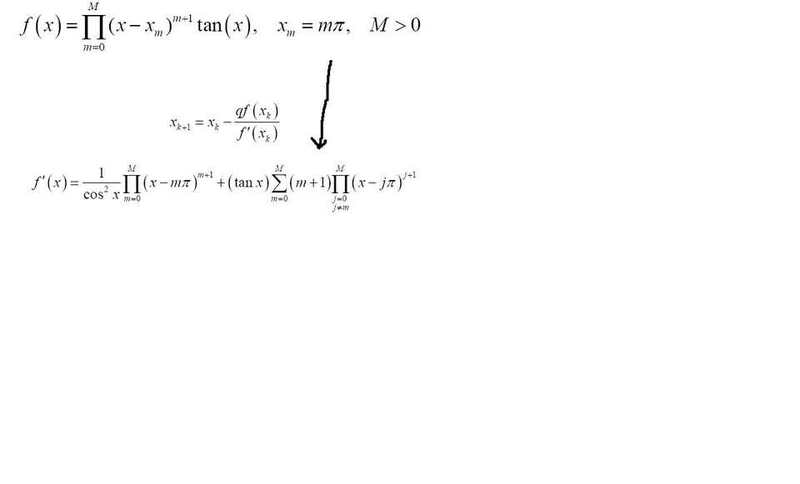# Derivative laws question numerical analysis

nhrock3i know the law (fg)'=f'g+fg'
but here there is epsilon
and it turns to someother sign i dont know what it says.

i cant understand the transition
?

## Answers and Replies

Mentori know the law (fg)'=f'g+fg'
but here there is epsilon
and it turns to someother sign i dont know what it says.

i cant understand the transition
?
There's no epsilon in what you posted. Are you talking about $\prod$? Note that this is upper-case pi, which is different from the constant $\pi$, lower-case pi.

Upper-case pi is similar to upper-case sigma $\sigma$, except that it is used for products rather than sums. here's an example using the product notation.
$$\prod_{m = 1}^5 m = 1 \cdot 2 \cdot 3 \cdot 4 \cdot 5 = 5! = 120$$

nhrock3
ok but why the derivative change epsilon to pi?

Mentor
What are you talking about? There is no epsilon.

Homework Helper
With epsilon do you mean the summation sign? If so that one is called capital sigma.

Taking the derivative of a function that is the product of two other functions yields two terms as you know, (fg)'=f'g+g'f. In our case we don't have a function consisting of the product of two functions but of a product of m functions.

$$\prod_{j=0}^m f_j=f_0f_1f_2 \cdot ... \cdot f_m$$

Now taking the derivative of that product yields:

$$\frac{d}{dx}\prod_{j=0}^m=\frac{d}{dx}(f_0f_1f_2 \cdot ... \cdot f_m)=f'_0f_1f_2 \cdot ... \cdot f_m+f_0f_1'f_2 \cdot ... \cdot f_m+...+f_0f_1f_2 \cdot ... \cdot f_m'$$

You can see that we get m terms that are added together and every term is the product of m terms. We can write this compactly as:

$$\sum_{i=0}^m \frac{df_i}{dx}\prod_{\substack{ j=0 \\ j \neq i}}^m f_j$$.

Last edited: